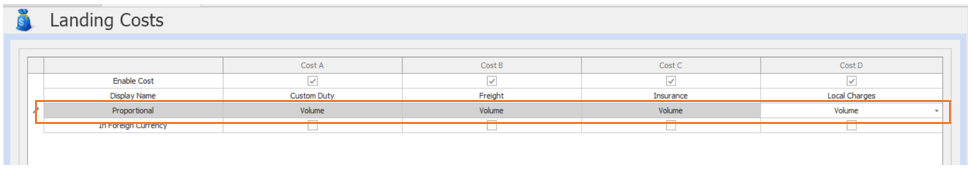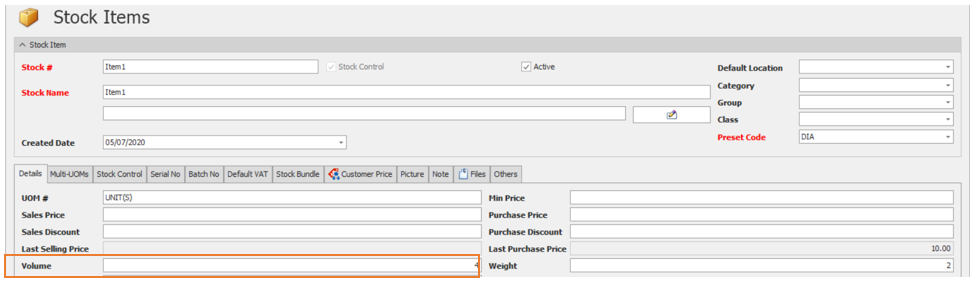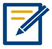# HOW LANDING COST BY VOLUME WORKS

Modified on Wed, 15 Jun 2022 at 11:32 PM

HOW LANDING COST BY VOLUME WORKS

Overview:

Another option for computing the Landing Cost is ‘By Volume’. Distribution of cost per Item purchased will be based on the volume. The bigger space taken up by an item, the higher the amount will be allocated.

Scenario:

Incorporate the Landed Cost in imported stocks and use volume as reference for allocating the total Landing Cost.

Procedure:

Enable ‘Landing Cost’.

1. Go to Purchases > Landing CostsSet the Proportional into ‘Volume’.Make sure that the Stocks has Volume to use this method.Item1 Volume: 4

Item2 Volume: 2

Application:

Issue a Purchase Invoice and use the Landing cost Calculator as shown below. Input the value of the landing cost.System will automatically compute the Value for Custom Duty, Freight, Insurance, and Local Charges.The calculation of proportion Volume is as below:

 Item1 Custom Duty(Qty x Volume)/Total (Qty x Volume) x Custom Duty = (2x4)/((2x4) +(2x2)) x PHP 10 = PHP 6.67 Insurance(Qty x Volume)/Total (Qty x Volume) x Insurance= (2x4)/((2x4) +(2x2)) x PHP 30= PHP 20 Freight(Qty x Volume)/Total (Qty x Volume) x Freight= (2x4)/((2x4) +(2x2)) x PHP 20= PHP 13.33 Local Charges (Qty x Volume)/Total (Qty x Volume) x Local Charges= (2x4)/((2x4) +(2x2)) x PHP 40= PHP 26.67

 Item2 Custom Duty(Qty x Volume)/Total (Qty x Volume) x Custom Duty = (2x2)/((2x4) +(2x2)) x PHP 10 = PHP 3.33 Insurance(Qty x Volume)/Total (Qty x Volume) x Insurance= (2x2)/((2x4) +(2x2)) x PHP 30= PHP 10 Freight(Qty x Volume)/Total (Qty x Volume) x Freight= (2x2)/((2x4) +(2x2)) x PHP 20= PHP 6.67 Local Charges (Qty x Volume)/Total (Qty x Volume) x Local Charges= (2x2)/((2x4) +(2x2)) x PHP 40= PHP 13.33

 NOTE: